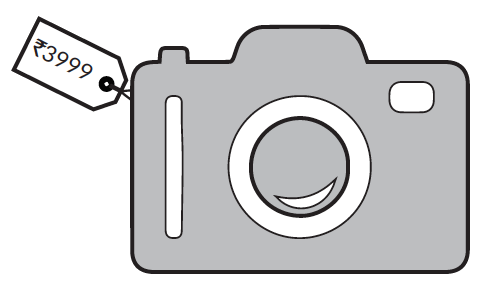# Discounts

Go back to  'Commercial Math'

Taxes and discounts are a natural extension of the application of percentages. These are probably the most frequent terms that come up during a conversation about shopping since they directly affect the amount of money involved. To understand tax and discount better, it is important to know the related terms.

## Marked price

Almost every item comes with a price tag attached to it (usually, by the manufacturer of the item). This price is called the marked price, abbreviated as MP.

Let’s try and understand this better using an example.

The price tag on a camera reads $$₹3999$$ but it is being sold for $$₹3900.$$The marked price of the camera is $$₹3999.$$ The selling price is $$₹3900.$$

## Discount

We come across banners like “$$50 \% \text{ OFF}$$” or “$$75 \% \text{ OFF}$$” on a frequent basis. These are usually in different places to attract customers like us and are usually put up in malls or big stores. The difference between the selling price and marked price is called discount.

Example: Nikhil visited Levi's and bought a shirt for $$₹2450.$$ If the price tag on the shirt read $$₹2940.$$ What is  the amount of discount offered by the store on the shirt?

The marked price of the shirt is $$₹2940$$ and the selling price is $$₹2450.$$

\begin{align}\text{Discount}& = \text{Marked Price} - \text{Selling Price} \\ & = ₹2940 - ₹2450 \\ & = ₹490 \end{align}

We can calculate the percentage of discount on the marked price.

\begin{align}\text{Discount Percentage}& = {\text{Discount} \over \text{Marked Price}} \times 100 \\\\ & = {490 \over 2940} \times 100 \\\\ & = 16.66 \% \end{align}

Note: Discount is calculated on selling price excluding taxes.

## Tips and Tricks

• Be careful when applying successive discounts. Discount percentages cannot be simply added. E.g. Two successive discounts of $$5 \%$$ is not equal to one discount of $$10 \%.$$
For example, an item that costs $$₹2000$$ is discounted successively by $$5 \%.$$ First discount value will be \begin{align} 2000 \times (5 \div 100) = ₹100. \end{align} Now the price has already reduced to $$₹1900.$$ The second discount will be \begin{align} 1900 \times (5 \div 100) = ₹95. \end{align} So the final price of the item is $$₹1805.$$ As you can see, this turns out to be slightly more expensive than a single discount of $$10 \%.$$
• Remember that discounts are calculated on the marked price (or listed price) of an item.

More Important Topics
Numbers
Algebra
Geometry
Measurement
Money
Data
Trigonometry
Calculus
More Important Topics
Numbers
Algebra
Geometry
Measurement
Money
Data
Trigonometry
Calculus
Learn from the best math teachers and top your exams

• Live one on one classroom and doubt clearing
• Practice worksheets in and after class for conceptual clarity
• Personalized curriculum to keep up with school

0